# Bloom Filter Data Structure: Implementation and Application

### What is Bloom Filter?

Bloom filter is a space-efficient probabilistic data structure that tells whether an element may be in a set or definitely is not. If we look up an item in the Bloom filter, we can get two possible results.

• The item is not present in the set: True negative.
• The item might be present in the set: Can be either a False positive or True positive.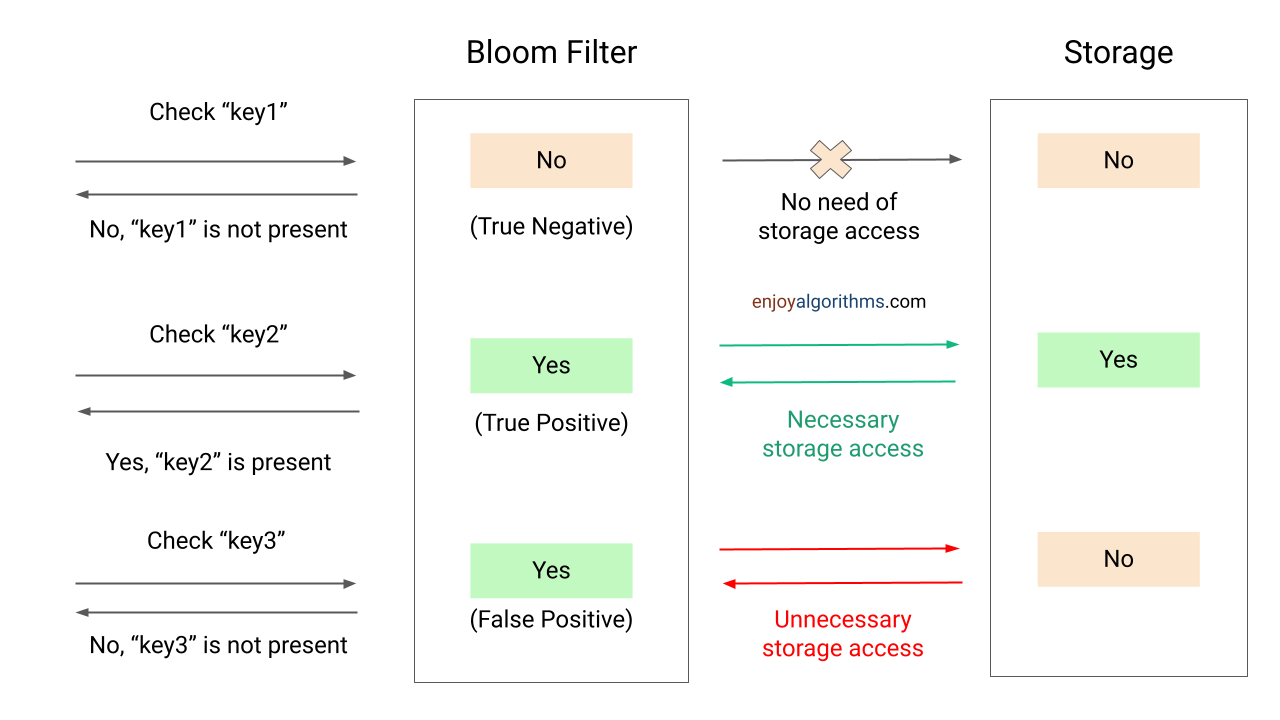### How does Bloom Filter work?

Suppose we want to compare two strings. Instead of storing the entire string, we compute the hash of the string and then compare the hashes. Computing hash and then comparing them takes O(1) time instead of the O(n) time to compare the strings directly. If both hashes are unequal, we can definitely say that strings are unequal. Otherwise, both strings may be equal!

One drawback of this method is the limited range of the hash function. The hash function h1 ranges from 0 to r1–1. We cannot uniquely identify more than r1 strings with this method, as at least two strings will have the same hash value. To compensate for this, we can use multiple hash functions.

Suppose we use k hash functions, h1, h2, …, hk, pass two strings through these hash functions, and compute their hashes. If all the hashes are identical for both strings, there is a very high probability that both strings are the same. On the other hand, even if one hash does not match, we can say that the strings are different.

Bloom filter is an array that stores 0s and 1s. In the image below, each cell represents a bit. The number below the bit is its index for a bloom filter of size 10.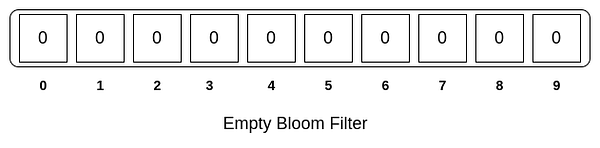### Inserting an item to the bloom filter

In order to add an element, we need to pass it through k hash functions. Bits are set at the index of the hashes in array. For example, we want to add the word “coding”. After passing it through three hash functions, we get the following results.

• h1(“coding”) = 125
• h2(“coding”) = 67
• h3(“coding”) = 19

Suppose that the size of our bloom filter is m = 10. We need to take mod of 10 for each of these values so that the index is within the bounds of the bloom filter. Therefore, indexes at 125%10 = 5, 67%10 = 7 and 19%10 = 9 have to be set to 1.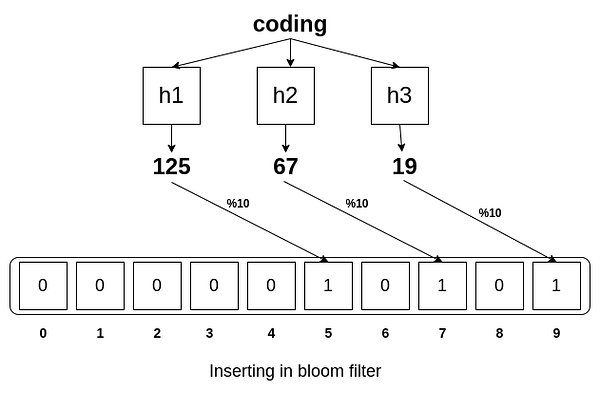### Testing membership of an item in Bloom filter

If we want to test the membership of an element, we need to pass it through same hash functions. If bits are already set for all these indexes, then this element might exist in the set. However, even if one index is not set, we are sure that this element is not present in the set.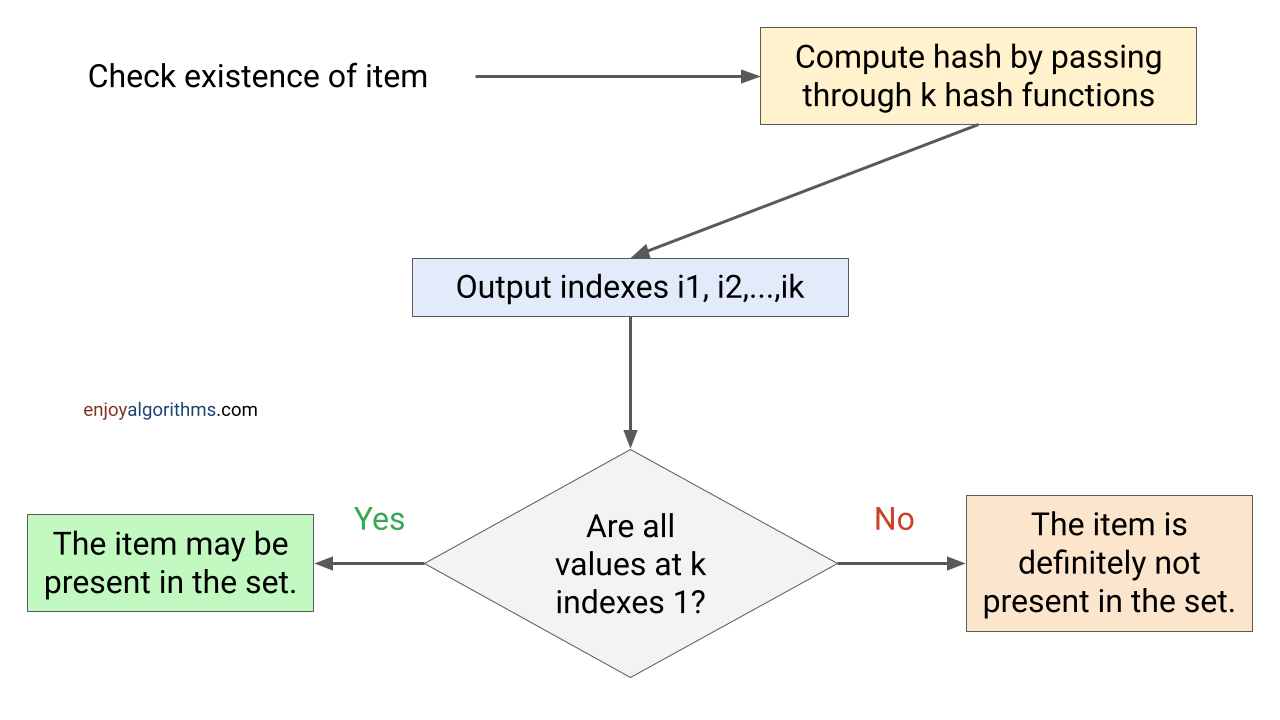Let’s say we want to check the membership of “cat” in our set. Furthermore, we have already added two elements, “coding” and “music”, to our set.

• Coding has the hash output {125, 67, 19} from the three hash functions, and as discussed above, the indexes {5, 7, 9} are set to 1.
• Music has the hash output {290, 145, 2} and the indexes {0, 2, 5} are set to 1.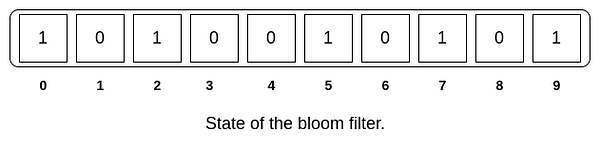We pass “cat” through the same hash functions and get the following results.

• h1(“cat”) = 233
• h2(“cat”) = 155
• h3(“cat”) = 9

So we check if the indexes {3, 5, 9} are all set to 1. As we can see, even though indexes 5 and 9 are set to 1, 3 is not. Thus we can conclude with 100% certainty that “cat” is not present in the set.

Now let’s say we want to check existence of “gaming” in our set. We pass it through same hash functions and get the following results.

• h1(“gaming”) = 235
• h2(“gaming”) = 60
• h3(“gaming”) = 22

We check if the indexes {0, 2, 5} are all set to 1. We can see that all of these indexes are set to 1. However, we know that “gaming” is not present in the set. So this is a false positive. Can we predict the probability of these false positives? Yes, and it is explained below.

Let n = number of elements, m = length of the bloom filter, k = number of hash functions.

Assume that the hash function selects each index with equal probability. The probability that a particular bit is not set to 1 by a specific hash function during the insertion of an element is 1-(1/m). If we pass this element through k hash functions, the probability that the bit is not set to 1 by any of the hash functions is (1 -(1/m))^k. After inserting inserted n elements, the probability that a particular bit is still 0 is (1 -(1/m))^(kn). Therefore the probability that it is one is 1 - (1 -(1/m))^(kn).

Here we want to test the membership of an element in the set. So each of the k array positions computed by the hash functions is 1 with a probability 1 -(1- (1/m))^(kn). The probability of all of them being 1, which would result in a false positive, is (1 -(1 -(1/m))^(kn))^k. This is also known as the error rate.

As we can see, if m tends to infinity, the error rate tends to zero. Another way to avoid a high false-positive rate is to use multiple hash functions.

### Bloom Filter Python Implementation

``````import mmh3 # murmurhash: is faster for blooms
import math

class BloomFilter(object):

def __init__(self, m, k):
self.m = m # size of bloom filter
self.k = k # number of hash functions
self.n = 0 # total count of the elemnts inserted in the set
self.bloomFilter = [0 for i in range(self.m)]

def _setAllBitsToZero(self):
self.n = 0
for i in self.bloomFilter:
self.bloomFilter[i] = 0

def getBitArrayIndices(self, item):
"""
hashes the key for k defined,
returns a list of the indexes which have to be set
"""

indexList = []
for i in range(1, self.k + 1):
indexList.append((hash(item) + i * mmh3.hash(item)) % self.m)
return indexList

"""
Insert an item in the filter
"""

for i in self.getBitArrayIndices(item):
self.bloomFilter[i] = 1

self.n += 1

def contains(self, key):
"""
returns whether item exists in the set or not
"""

for i in self.getBitArrayIndices(key):
if self.bloomFilter[i] != 1:
return False
return True

def length(self):
"""
returns the current size of the filter
"""

return self.n

def generateStats(self):
"""
Calculates the statistics of a filter
Probability of False Positives, predicted false positive rate, n, m, k.
"""

n = float(self.n)
m = float(self.m)
k = float(self.k)
probability_fp = math.pow((1.0 - math.exp(-(k*n)/m)), k)

print("Number of elements entered in filter: ", n)
print("Number of bits in filter: ", m)
print("Number of hash functions used: ", k)

print("Predicted Probability of false positives: ", probability_fp)
print("Predicted false positive rate: ", probability_fp * 100.0, "%")

def reset(self):
"""
Resets the filter and clears old values and statistics
"""

self._setAllBitsToZero()``````

### Bloom Filter Visualization With Example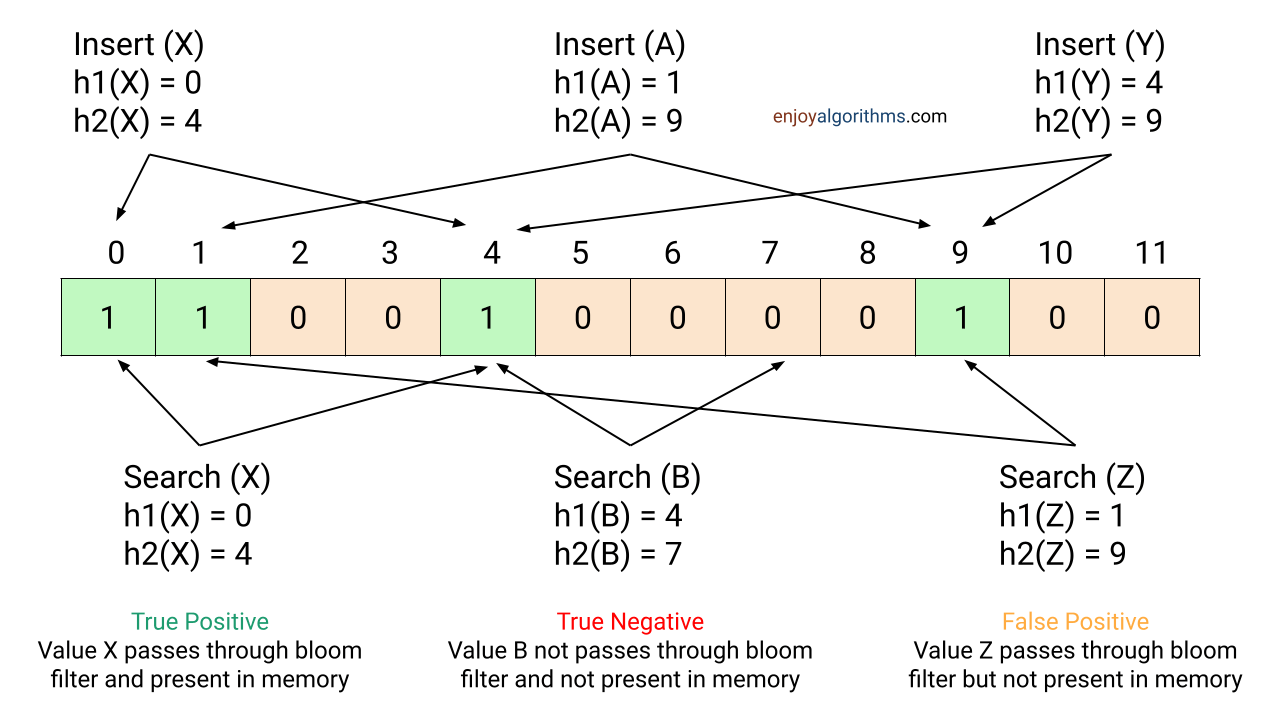### Bloom Filter Applications

Bloom filters can be used as the first layer of filtering. The advantage is that they require constant space. They can be helpful where we want to know if something is definitely not present or possibly present somewhere. For example, we want to query a large database from a rotating hard drive that is slow to read. Suppose there is a high probability that the thing we want to query is not present in the database, then before querying, we can check for this item in the bloom filter. We can avoid the expensive query if the bloom filter says that this element is definitely not present in the database.

• Facebook uses bloom filters to avoid one-hit wonders. One-hit wonders are web objects requested by users just once. Using a Bloom filter to detect the second request for a web object and caching it only on its second request prevents one-hit wonders from entering the local storage. For example, searching for “coding” often can be stored in the local storage. However, if you search for something only once, like “giraffe”, it should not be stored in the local storage as it will be a one-hit-wonder.

Using an LRU Cache here will remove some older but frequent results from the local storage for one-hit wonders, negatively affecting the user experience.

• Medium uses Bloom filters in its Recommendation module to avoid showing those posts that the user has already seen.

### Summary

A Bloom filter is a data structure designed to tell rapidly and memory-efficiently whether an element is present in a set. The tradeoff is that it is probabilistic; it can result in False positives. Nevertheless, it can definitely tell if an element is not present. Bloom filters are space-efficient; they take up O(1)space, regardless of the number of items inserted as the bloom filter size remains the same. However, their accuracy decreases as more elements are added. Insert, and lookup operations are O(1) time.

Enjoy learning, Enjoy algorithms!

☆ 16-Week Live DSA Course
☆ 10-Week Live DSA Course